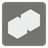Fused Cubes
Moderate
English JA

This is a mission to calculate the volume of objects that combines cubes.

You are given a list of cube details (tuple of 4 integers: X coordinate, Y coordinate, Z coordinate, edge length).

• Each coordinate is the minimum value.
• All edges parallel to the coordinate axes.

If the cube share the part of another cube or touch with the face of another cube, they are considered as one object.
You should return a list (or iterable) of the volumes of all objects.

Example:

```sorted(fused_cubes([(0, 0, 0, 3), (1, 2, 2, 3)])) ==        # fused
sorted(fused_cubes([(0, 0, 0, 3), (1, 3, 2, 3)])) ==        # touch with faces
sorted(fused_cubes([(0, 0, 0, 3), (1, 3, 3, 3)])) == [27, 27]   # touch with edges```input: A list of tuples of 4 integers.

output: A list (or iterable) of integers.